# Modelling of two phase flow in packed bed (continued)

• casualguitar
Hi Chet,

Unfortunately the molar flow error didnt fix the output, although it did change it somewhat.

The molar flow out of a tank should have been defined as the below. It was previously this so I must have changed it somewhere along the way:
\begin{equation}
\dot{m}_j = \dot{m}_{j-1} + \frac{\rho_m \Delta z A_c}{T_g}\frac{dT}{dt} - (M_{CO_2}''*A_s)
\end{equation}

Further up in the code ##m_j## is defined as:
\begin{equation}
m_j = \frac{P}{RT_g}\epsilon A_C\Delta z
\end{equation}

So I now have:
\begin{equation}
\dot{m}_j = \dot{m}_{j-1} + \frac{\rho_m \Delta z A_c}{T_g}\frac{dT}{dt} - (M_{CO_2}''*A_s)
\end{equation}

which I've implemented as:
\begin{equation}
\dot{m}_j = \dot{m}_{j-1} + \frac{m_j \epsilon}{T_g}\frac{dT}{dt} - (M_{CO_2}''*A_s)
\end{equation}

Just posting this here in case I've done anything crazy there

Hi Chet, looking further into the CO2 desublimation rate function, these are plots of temperature versus sublimation pressure (the gas-solid curve), and then temperature versus desublimation rate for a range of ##k_i## values, and ##y_{CO2}## = 0.2 (as it is in Tuinier et al).

Note that for the sublimation pressure curve, for temperature values above the triple point temperature (216K), i've used the liquid-vapour curve. Is there an alternative here? We could possibly say: if T is less than the triple point temperature, use the sublimation pressure curve, if T is greater than the triple point temeprature, let sublimation pressure = some large number?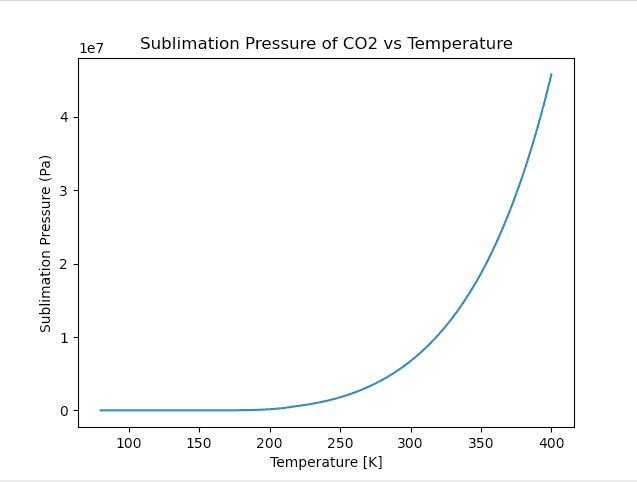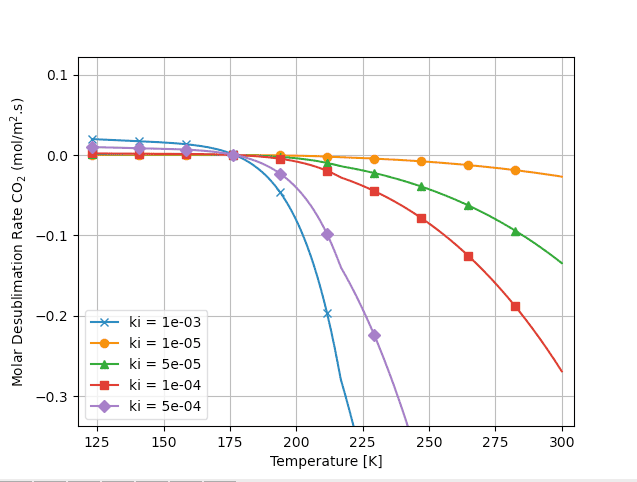Edit: just noting the simulation breaks if I let psubco2 = 1000000 for T > triple point temerpature

Hi Chet, one more finding:

I ran a range of ##k_{CO2}## values as they have a very significant effect on all of the output. Here they are:

##k_{CO2}## = ##5*10^-4##: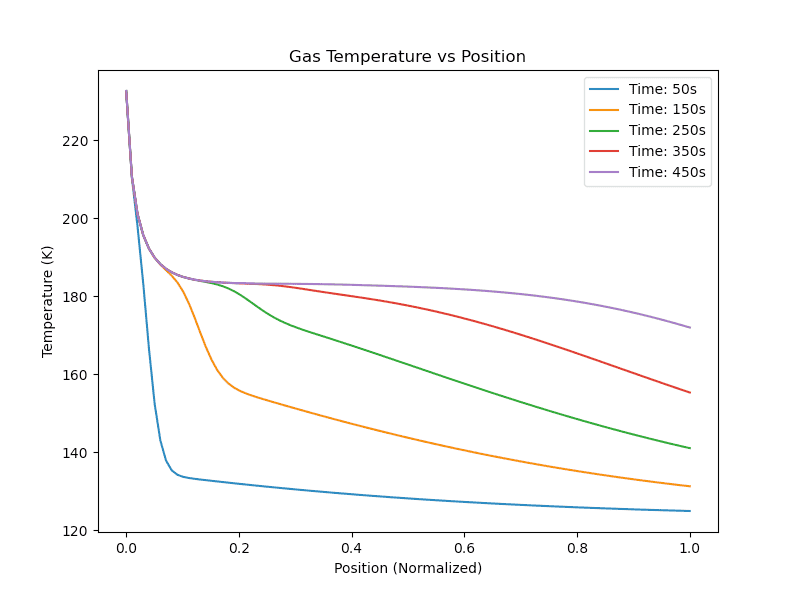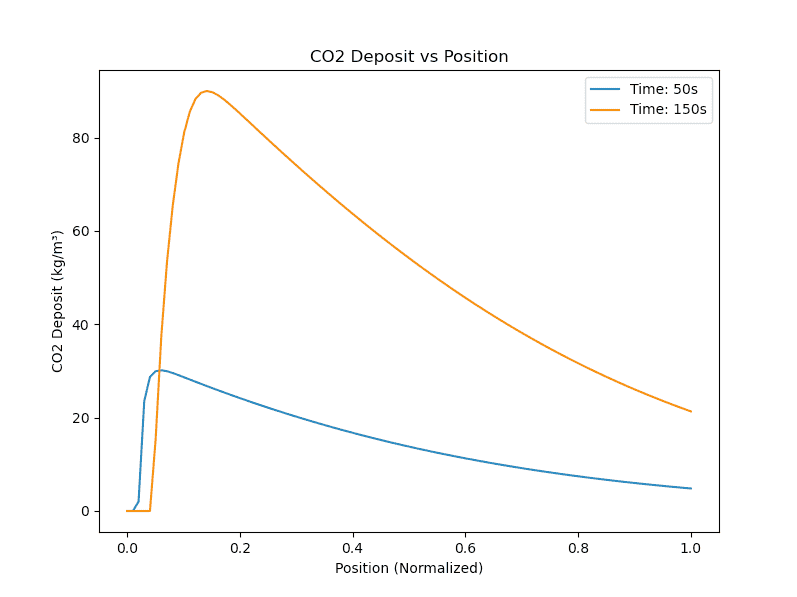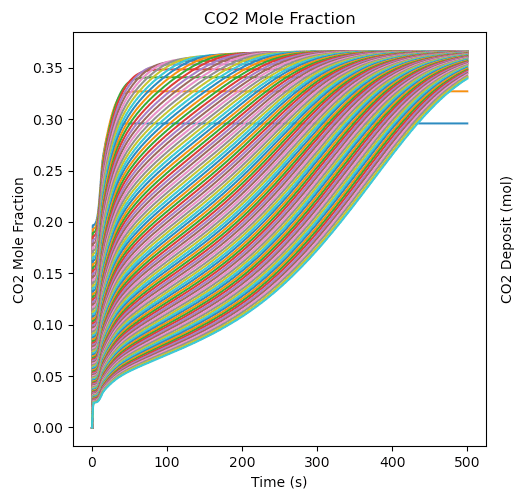##k_{CO2}## = ##5*10^-5##: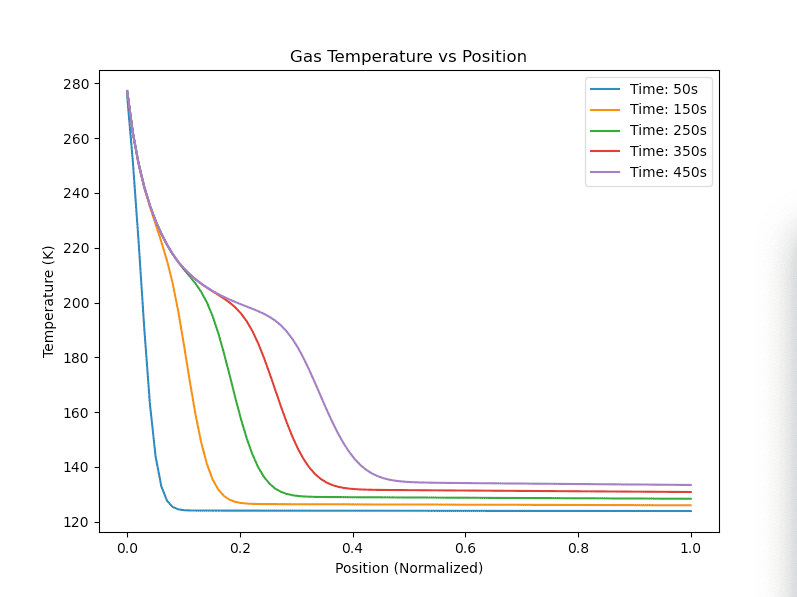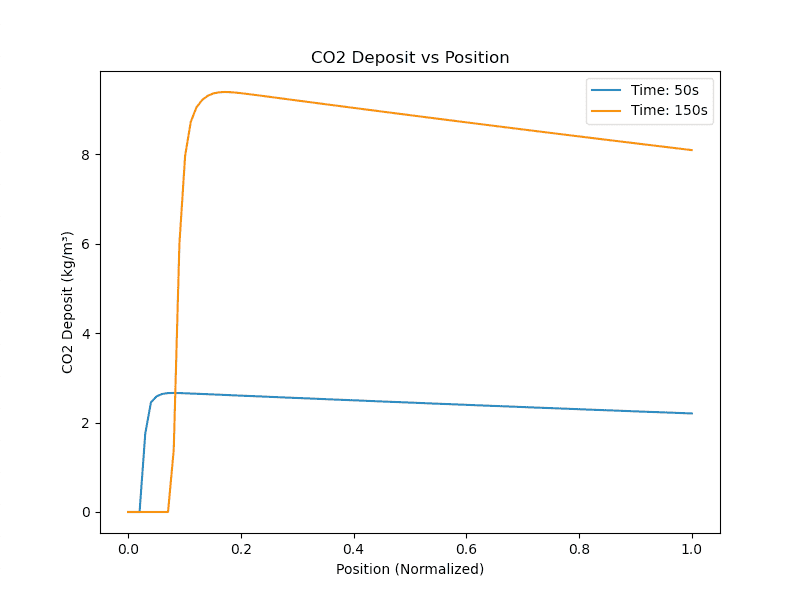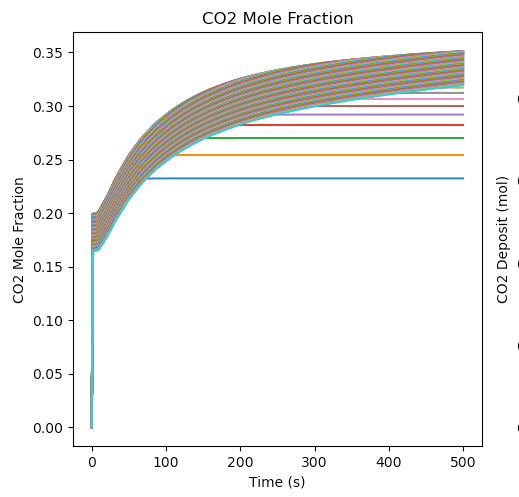##k_{CO2}## = ##5*10^-6##: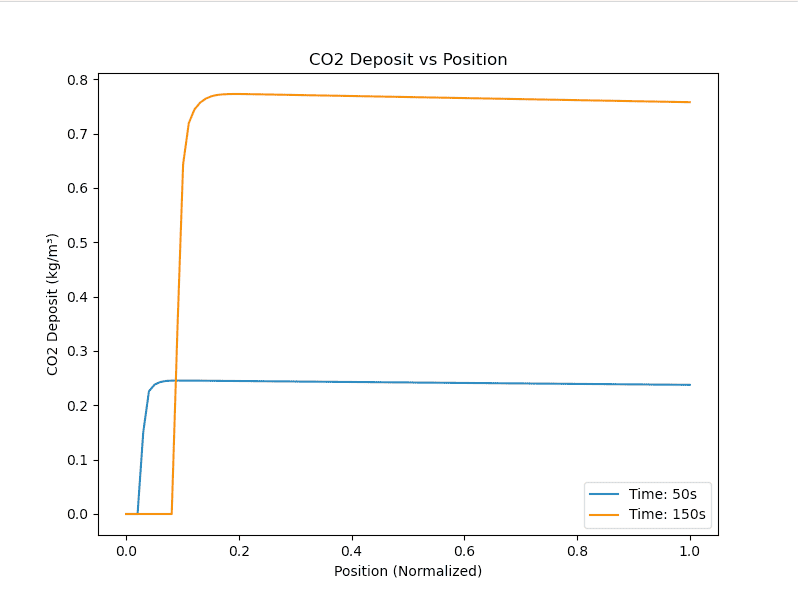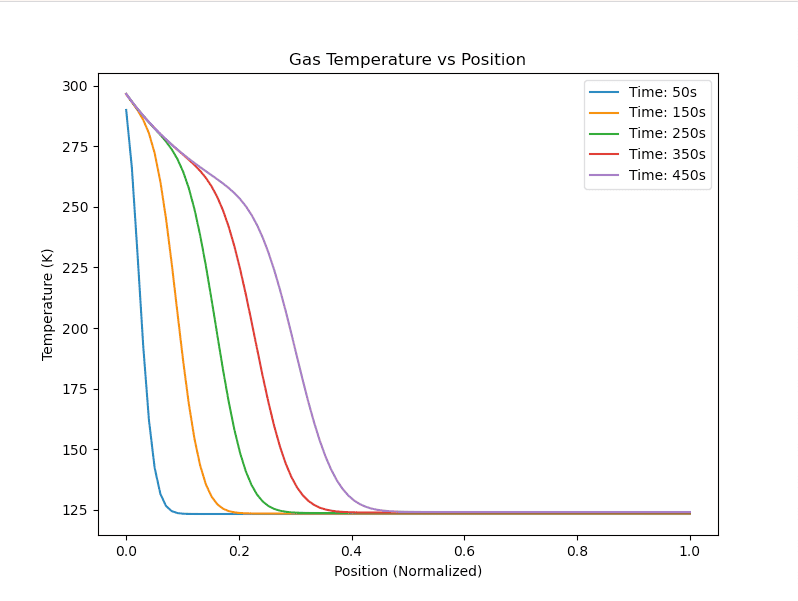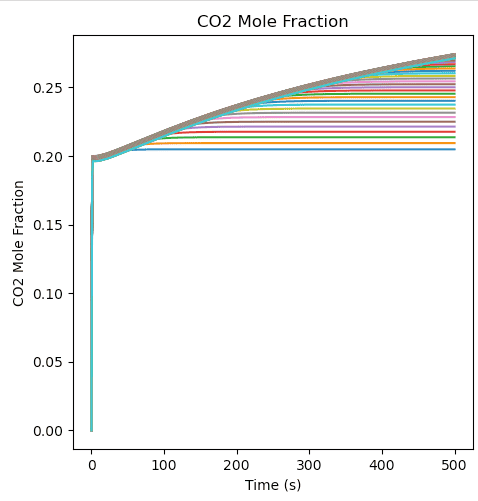So yes again you were right with your original thought that CO2 sublimation isn't working correctly. Actually I've printed the CO2 sublimation pressure values versus temperature and as temperature gets to high values the sublimation pressure does too, meaning that we should see high sublimation rates of CO2, but we dont (the CO2 deposition plot is horizontal at high temperatures).

#### Attachments

My apologies for the long-winded posts (my head is a bit fried!). The first plot below is completely at odds with the second one is it not? These plots are from the same simulation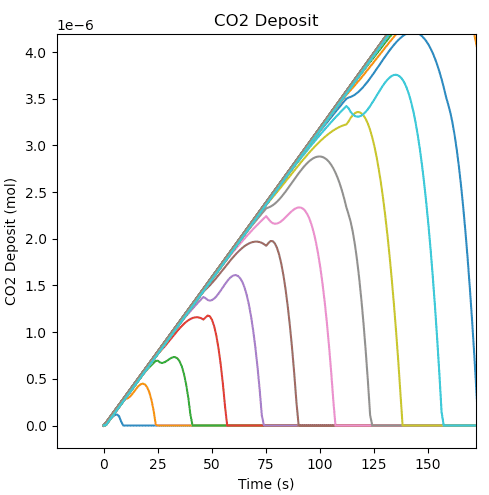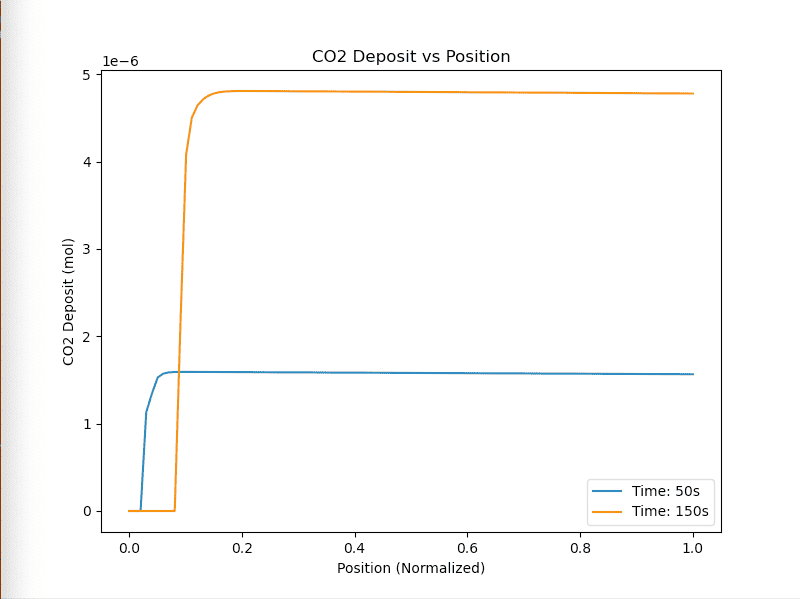Hi Chet,

Output is looking better now. Only issue really is that we're producing a lot more solid CO2. The profiles look ok though.

I reverted back to an older approach we had, where we used something like their "fudge factor". I also put in the previous correlation for ##k_{CO2}##.

The fudge factor looks like this and only applies for sublimation (not desublimation):
if ##P*y_{CO2}## < ##P_{sub,CO2}##:
##M_{CO2}''## = ##M_{CO2}''##*##(\frac{M_{CO2}}{M_{CO2}+0.1})##

Tuinier uses 0.1 as their fudge factor. I'm using 0.1 also currently. We previously also discussed converting this 0.1 to a value suitable for us (different units), like this:
factor = 0.1*1000*A_C*dz/mW_CO2

As we're now calculating ##k_{CO2}##, we can no longer use this as a tuning parameter, unless I'm wrong. I'm wondering if we can instead use this fudge factor to tune the amount of CO2 produced?

Here's the output from the above. Note the output is a bit rough looking because I have used n=15. The the simulation takes a lot longer to run now so I'll increase this again once we're happy with the output:

Gas temperature profile shows the constant temperature zone: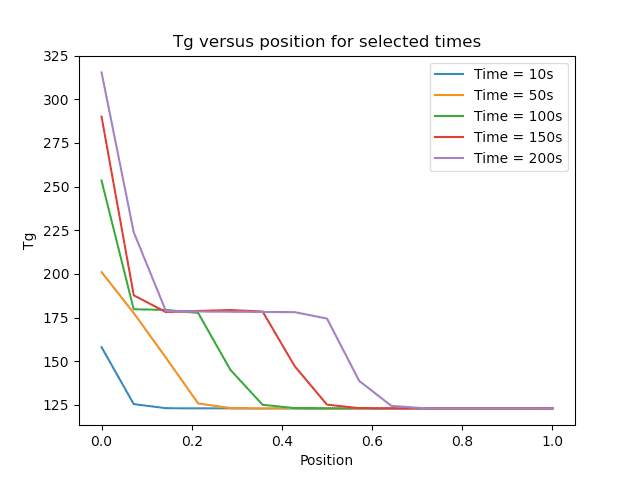The amount of CO2 on the bed in kg/m3. Tuinier et al had values closer to 50kg/m3 for 50s and 150s: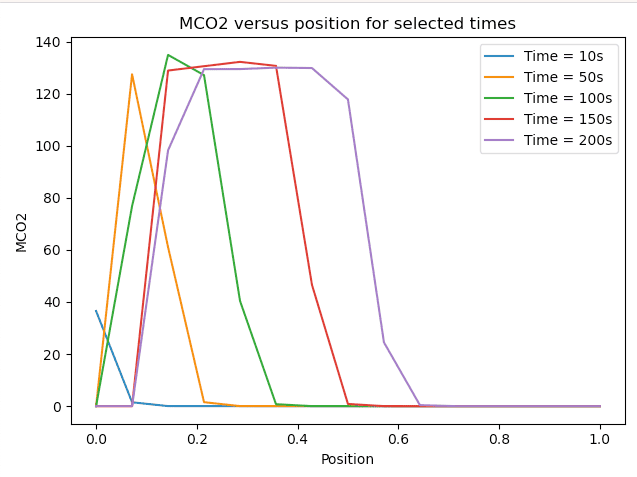Lastly, ##y_{CO2}## for each position. Each position does level out at 0.2 which is the inlet concentration. There is a bit of fluctuation but this is possibly down to the sublimation process (I'll check this):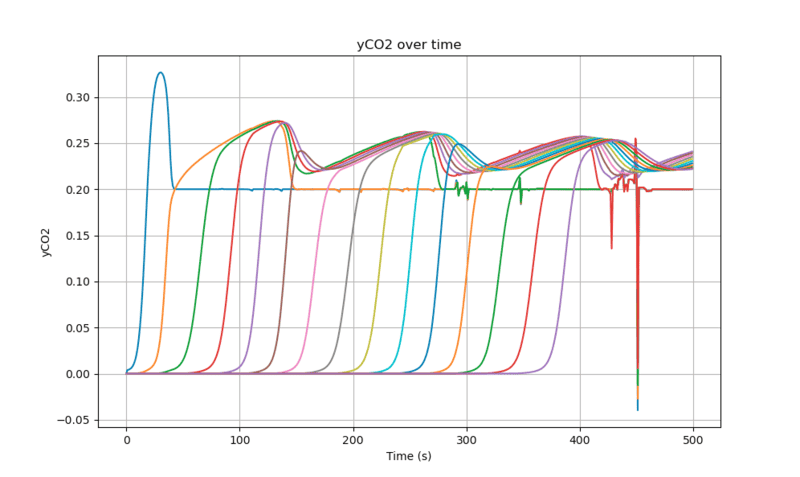If this looks ok to you, do you think it is reasonable to use the "factor" to tune the amount of CO2 on the bed to Tuinier et al?

EDIT: If I take out the fudge factor and run it, the output looks like it did before (incorrect). I didn't think the fudge factor would have such an impact!

#### Attachments

Last edited:
Hi Chet,

Unfortunately the molar flow error didnt fix the output, although it did change it somewhat.

The molar flow out of a tank should have been defined as the below. It was previously this so I must have changed it somewhere along the way:
\begin{equation}
\dot{m}_j = \dot{m}_{j-1} + \frac{\rho_m \Delta z A_c}{T_g}\frac{dT}{dt} - (M_{CO_2}''*A_s)
\end{equation}

Further up in the code ##m_j## is defined as:
\begin{equation}
m_j = \frac{P}{RT_g}\epsilon A_C\Delta z
\end{equation}

So I now have:
\begin{equation}
\dot{m}_j = \dot{m}_{j-1} + \frac{\rho_m \Delta z A_c}{T_g}\frac{dT}{dt} - (M_{CO_2}''*A_s)
\end{equation}

which I've implemented as:
\begin{equation}
\dot{m}_j = \dot{m}_{j-1} + \frac{m_j \epsilon}{T_g}\frac{dT}{dt} - (M_{CO_2}''*A_s)
\end{equation}

Just posting this here in case I've done anything crazy there
I think there should be an ##\epsilon## in the term of Eqn.1 and no ##\epsilon## in the term of Eqn.4. That assumes that Ac is the cross sectional area of the empty column.

Last edited:
Hi Chet, looking further into the CO2 desublimation rate function, these are plots of temperature versus sublimation pressure (the gas-solid curve), and then temperature versus desublimation rate for a range of ##k_i## values, and ##y_{CO2}## = 0.2 (as it is in Tuinier et al).

Note that for the sublimation pressure curve, for temperature values above the triple point temperature (216K), i've used the liquid-vapour curve. Is there an alternative here? We could possibly say: if T is less than the triple point temperature, use the sublimation pressure curve, if T is greater than the triple point temeprature, let sublimation pressure = some large number?
View attachment 326637
No. You should be using the actual curve, although there is melting at the triple point (along with a latent heat effect) and, above the triple point, you should more properly be using the liquid vapor pressure.

Note that, at the triple point, the equilibrium vapor pressure is 5 bars, and at the critical point, the equilibrium vapor pressure is 74 bars.

Please plot the equilibrium vapor pressure on a logarithmic scale.
View attachment 326638

Edit: just noting the simulation breaks if I let psubco2 = 1000000 for T > triple point temerpature

My apologies for the long-winded posts (my head is a bit fried!). The first plot below is completely at odds with the second one is it not? These plots are from the same simulation
View attachment 326649

View attachment 326650
What happened to evaporation of the deposits? I assume these values are in each tank?

Hi Chet,

Output is looking better now. Only issue really is that we're producing a lot more solid CO2. The profiles look ok though.

I reverted back to an older approach we had, where we used something like their "fudge factor". I also put in the previous correlation for ##k_{CO2}##.

The fudge factor looks like this and only applies for sublimation (not desublimation):
if ##P*y_{CO2}## < ##P_{sub,CO2}##:
##M_{CO2}''## = ##M_{CO2}''##*##(\frac{M_{CO2}}{M_{CO2}+0.1})##

Tuinier uses 0.1 as their fudge factor. I'm using 0.1 also currently. We previously also discussed converting this 0.1 to a value suitable for us (different units), like this:
factor = 0.1*1000*A_C*dz/mW_CO2

As we're now calculating ##k_{CO2}##, we can no longer use this as a tuning parameter, unless I'm wrong. I'm wondering if we can instead use this fudge factor to tune the amount of CO2 produced?

Here's the output from the above. Note the output is a bit rough looking because I have used n=15. The the simulation takes a lot longer to run now so I'll increase this again once we're happy with the output:

Gas temperature profile shows the constant temperature zone:
View attachment 326677

The amount of CO2 on the bed in kg/m3. Tuinier et al had values closer to 50kg/m3 for 50s and 150s:
View attachment 326678

Lastly, ##y_{CO2}## for each position. Each position does level out at 0.2 which is the inlet concentration. There is a bit of fluctuation but this is possibly down to the sublimation process (I'll check this):
View attachment 326679

If this looks ok to you, do you think it is reasonable to use the "factor" to tune the amount of CO2 on the bed to Tuinier et al?

EDIT: If I take out the fudge factor and run it, the output looks like it did before (incorrect). I didn't think the fudge factor would have such an impact!
What the fudge factor does is prevent M from going negative. The amount of CO2 on the bed should always be positive.

I'm concerned about those much higher depositions of CO2 per unit area than the comparison calculation.

FWIW
https://en.wikipedia.org/wiki/Darcy's_law
https://www.wikiwand.com/en/Buckley–Leverett_equation
https://en.wikipedia.org/wiki/Black-oil_equations

The Constitutive relationships need to be assumed or defined to include all fluid properties, such as;
density, viscosity, and thermal conductivity, which may vary with saturation and phase distribution.

In another unrelated question on energy conversion for work done on a conveyor belt, I postulated if one desires maximum power transfer or energy thruput or MPT or max. energy flow with a lossy interface (eddy currents) the minimum energy lost is 50% due to the MPT theorem. I also stated, in theory, if loss resistance is constant , time is irrelevant to efficiency and friction is irrelevant to losses. (not true here for nonlinear velocity related losses)

However, various time constants can be linearized from these complex models for certain assumptions.

FWIW ( TL;DR both threads ) so just a comment.

•casualguitar
Hi Chet, my apologies for the late response. Here's the vapour-solid (sublimation) curve, where for temperatures above the triple point temperature I've used the vapour-liquid curve. At the triple point temperature, the vapour pressure is 5 bar. Standard scale and log scale:

Standard scale: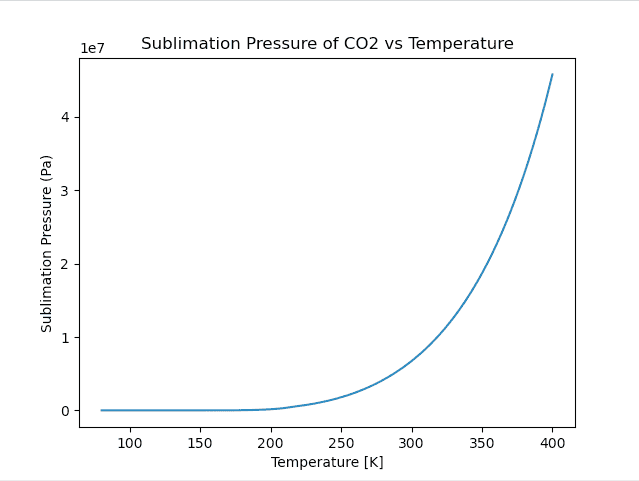Log scale: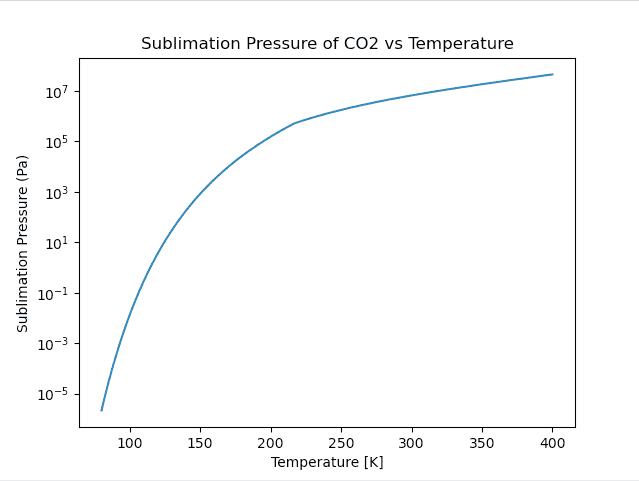What happened to evaporation of the deposits? I assume these values are in each tank?
If you're asking if the first plot there is for each tank then yes that's correct

I'm concerned about those much higher depositions of CO2 per unit area than the comparison calculation.
##k_{CO2}## seems to be the main variable impacting the max amount of CO2 desposited on the bed. My thought is that maybe my ##K_{CO2}## calculation is incorrect

Printing the Reynolds number and the ##K_{CO2}## numbers for the simulation, the Reynolds number seems to be pretty constant at around 2500. ##K_{CO2}## ranges from abour 0.2 to 0.3 which I think is in agreement with the plot below: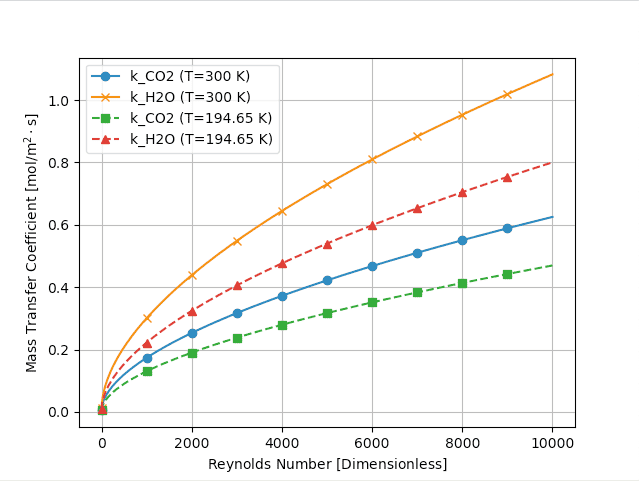Here is the equation I'm using to calculate ##k_{CO2}##:

##k_{CO2}= (2.19Re^{1/3} + 0.78*Re^{0.619})*Sc^{1/3} * D_{CO2,N2}*(1-\epsilon)/d_p##

Where:
##Sc_{CO2} = \frac{\mu_{CO2}}{\rho_{CO2}*D_{CO2,N2}}##

Where ##\mu## is in ##Pa.s##, ##\rho## is ##\frac{kg}{m3}##, ##d_p## is in ##m##

and ##D_{CO2,N2}## = ##0.144*10^4## ##m^2/s##

The units clearly don't match up here. I know we previously had a molar density term in here. Let me go through this ##k_i## derivation from the start tomorrow

Hi Chet, my apologies for the late response. Here's the vapour-solid (sublimation) curve, where for temperatures above the triple point temperature I've used the vapour-liquid curve. At the triple point temperature, the vapour pressure is 5 bar. Standard scale and log scale:

Standard scale:
View attachment 326837

Log scale:
View attachment 326838

If you're asking if the first plot there is for each tank then yes that's correct

##k_{CO2}## seems to be the main variable impacting the max amount of CO2 desposited on the bed. My thought is that maybe my ##K_{CO2}## calculation is incorrect

Printing the Reynolds number and the ##K_{CO2}## numbers for the simulation, the Reynolds number seems to be pretty constant at around 2500. ##K_{CO2}## ranges from abour 0.2 to 0.3 which I think is in agreement with the plot below:
View attachment 326839

Here is the equation I'm using to calculate ##k_{CO2}##:

##k_{CO2}= (2.19Re^{1/3} + 0.78*Re^{0.619})*Sc^{1/3} * D_{CO2,N2}*(1-\epsilon)/d_p##

Where:
##Sc_{CO2} = \frac{\mu_{CO2}}{\rho_{CO2}*D_{CO2,N2}}##

Where ##\mu## is in ##Pa.s##, ##\rho## is ##\frac{kg}{m3}##, ##d_p## is in ##m##

and ##D_{CO2,N2}## = ##0.144*10^4## ##m^2/s##

The units clearly don't match up here. I know we previously had a molar density term in here. Let me go through this ##k_i## derivation from the start tomorrow
Shouldn't the units of k be m/s?

Shouldn't the units of k be m/s?
Thats correct. I checked the ##k_{CO2}## units and it seems to be fine. I also printed out all of the solution data to csv/excel to see if there was anything unusual happening in the data that isn't being plotted and there wasn't.

I did find something interesting though - the bed properties have an obviously significant effect on the output, but some properties are not clearly defined in Tuinier et al.

Tuinier bed data:
- 'glass' particles
- 4.04mm diamter spherical particles
- particle density = 2547 kg/m3
- inner diameter x length of bed = 35mm x 300mm

Missing values required for the simulation are:
- thermal conductivity of the particles (doesn't have a significant effect on the output, just used in ##U_b## calculation)
- heat capacity of the particles
- molecular weight of the particles (has a very significant effect on the output, I found an approx value for this online)
- bed voidage (also has a significant effect on the output)

So I found the PhD thesis that this publication is from. It doesn't mention the molecular weight/heat capacity/thermal conductivity. It does give a porosity value of 0.7 for one simulation though (its not the same simulation, but its a very similar simulation to the one in this paper). Nevertheless when I put 0.7 into the model I get similar output to what they got (the max values are similar, the trends are similar, and the width of the CO2 plugs are similar)

Our output: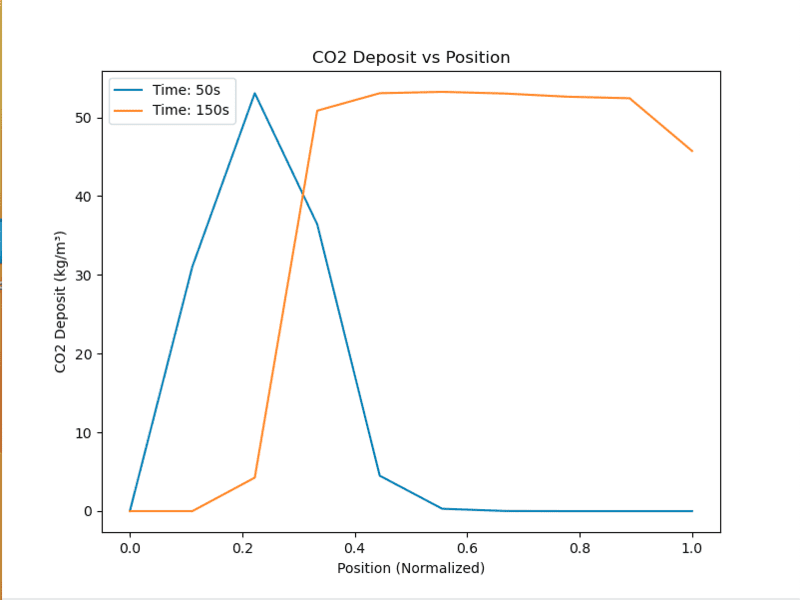Tuinier et al output: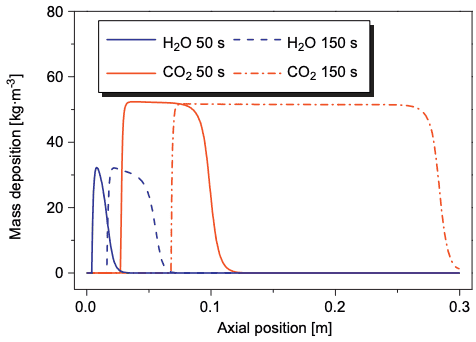So maybe they used voidage = 0.7?

Just note I'm using n=10 so that I can run simulations quickly. The output doesnt change much for higher n other than look smoother

The temperature profiles also look generally ok: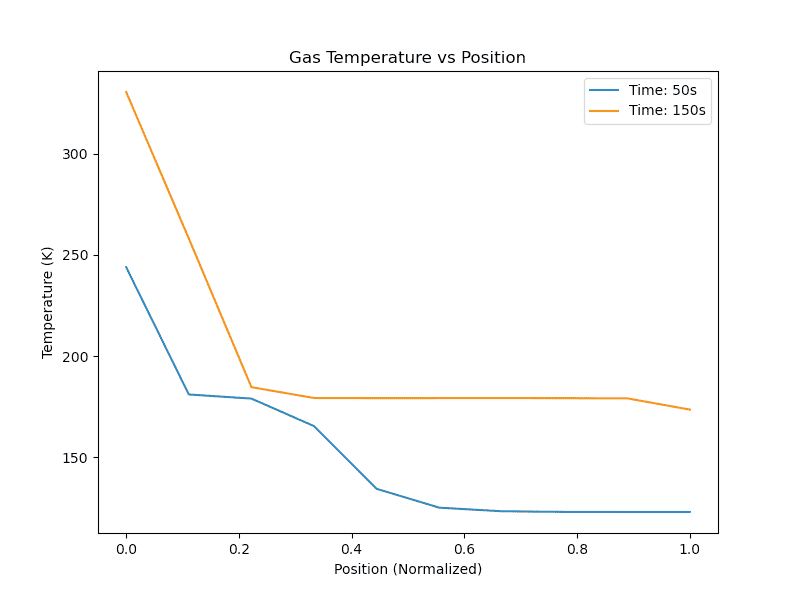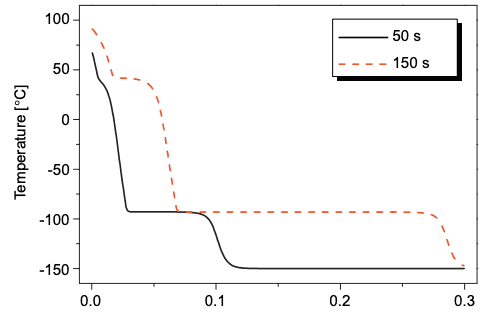What are the rules to fine-tuning models? Which parameters here can be used as "tuning" ones if any?

Nevertheless when I put 0.7 into the model I get similar output to what they got (the max values are similar, the trends are similar, and the width of the CO2 plugs are similar)

A (very) quick Google search found packing densities from 6% to 78%, which puts porosity at 94% to 22%.

Here is one paper that shows packing density below 60%.
https://www.osti.gov/servlets/purl/4518893

And one than shows the packing range is between 6% and 74%.
(https://mathworld.wolfram.com/SpherePacking.html)

It looks like of case of "You pays yo' money an' takes your choice."

Cheers,
Tom

p.s. Even though the subject is WAY outside my field, I have found the thread interesting enough to have followed from its start. It is not often that we see two experts collaborating here.

Last edited:
•casualguitar
Shouldn't the units of k be m/s?
Just in addition - in order to credibly use this model, I suppose I would need to add the ##H_2O## ODEs back in and tune the model with these included? Then remove them after tuning

Just in addition - in order to credibly use this model, I suppose I would need to add the ##H_2O## ODEs back in and tune the model with these included? Then remove them after tuning
This is a matter of your best judgment.

This is a matter of your best judgment.
I can add these back in to see if changing the porosity 0.7 in the above plots was just a lucky coincidence. If it wasn't a coincidence then we should see that the ##H_2O## buildup plots are also approximately correct

In regards to fine tuning the above plots (post #398), can we similarly use the number of tanks here to tune to Tuinier et al? Every other variable seems to be tied up in correlations

I'll work towards the above now unless you think otherwise

I can add these back in to see if changing the porosity 0.7 in the above plots was just a lucky coincidence. If it wasn't a coincidence then we should see that the ##H_2O## buildup plots are also approximately correct
oIsn't the porosity 0.3?
In regards to fine tuning the above plots (post #398), can we similarly use the number of tanks here to tune to Tuinier et al? Every other variable seems to be tied up in correlations
I think so. This all is not exact representation of reality, right? It's a model approximation.

I think so. This all is not exact representation of reality, right? It's a model approximation.
Thats true, I'll use the tank number then

oIsn't the porosity 0.3?
Hmm. The paper doesn't seem to provide a porosity value. I found the PhD thesis that this publication came from online. In the section about this model, they don't seem to give the porosity value, but they do give the porosity for a number of other very similar simulations (using the same model as is in the paper), and the porosity value they use is ##0.7##. In addition, when I use their value of 0.7 I get those plots in post #398 that are very similar to theirs. Here's the thesis: https://pure.tue.nl/ws/files/3606277/719418.pdf

If 0.7 is reasonable, then all that remains is to increase n until we (hopefully) get the same output as Tuinier

Here are the CO2 deposition and temperature profile plots with n=100, not including the ##H_2O## ODEs:

CO2 deposition plot comparisons (our model, Tuinier model):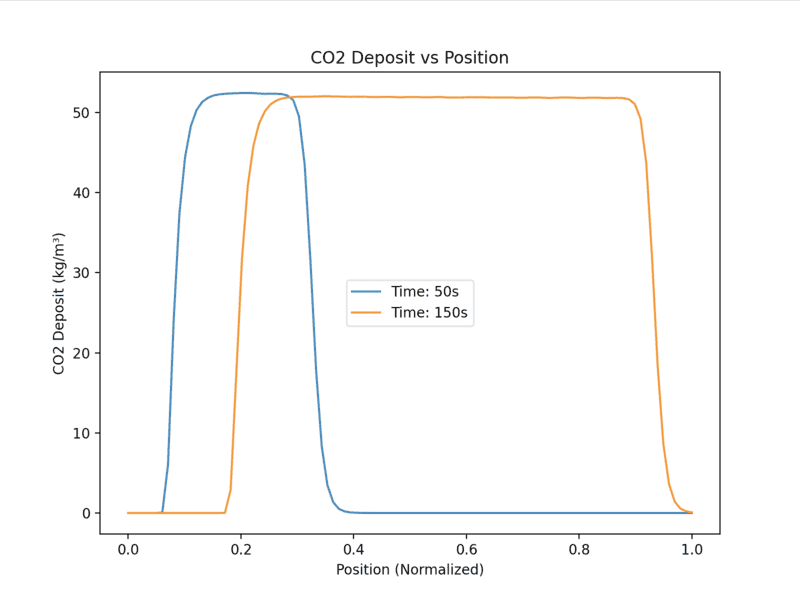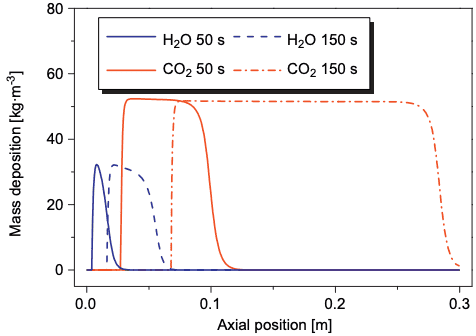Temperature profiles: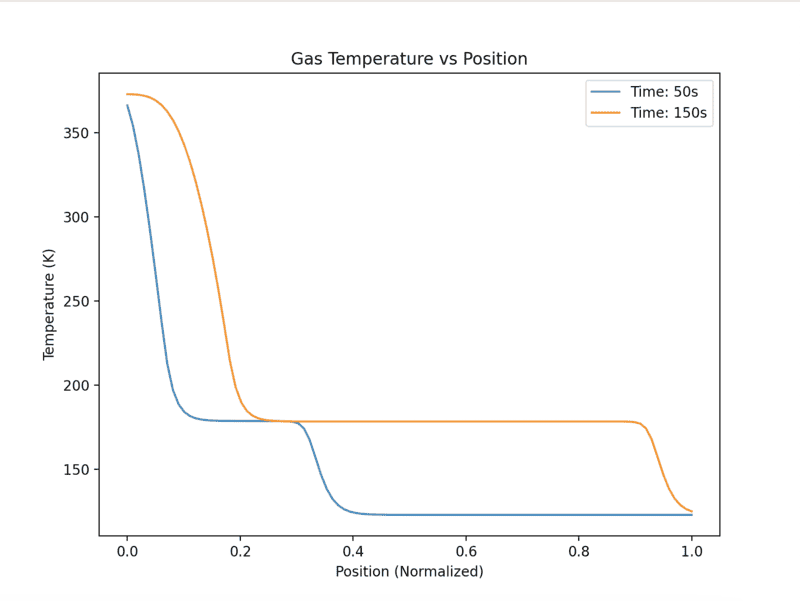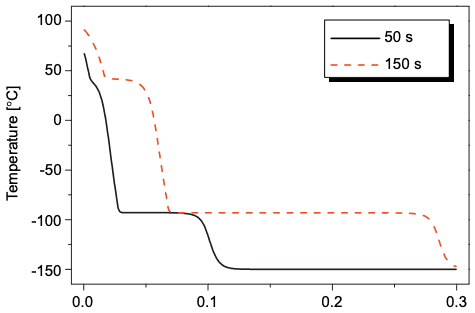The deposition profiles are very similar. The temperature profile from our model is missing that first almost constant temperature zone at around 40C. I'm not sure what that is. Presumably its the water freezing but the temperature seems to be a bit high. Besides this it looks pretty good

P.s. My apologies for not providing the same units as Tuinier. I'll get this included in the next version that will have the ##H_2O## ODEs included

Last edited:
I think so. This all is not exact representation of reality, right? It's a model approximation.

Here is the output after adding ##H_2O## back in. It looks good in my opinion. I ran for n=50 because n=100 was going to take too long (I'll rerun now at n=100 to get smoother plots)

Gas temperature: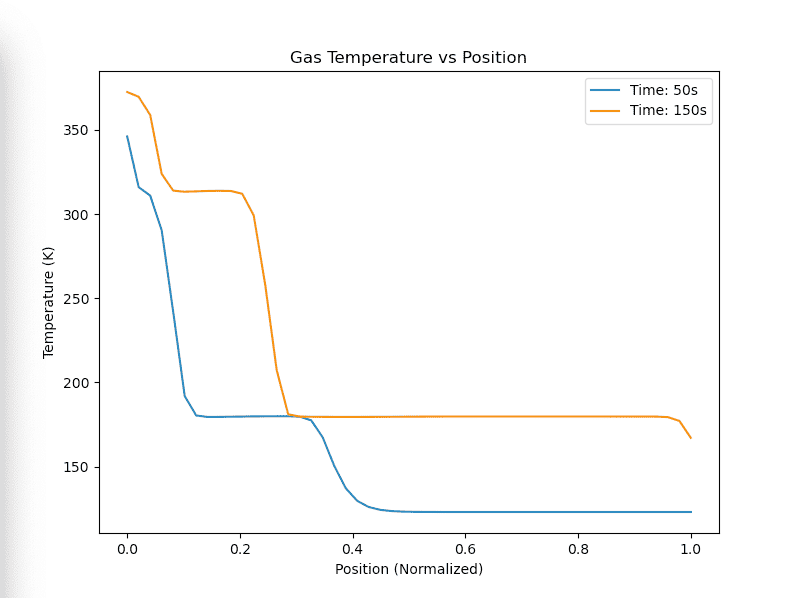CO2 deposition: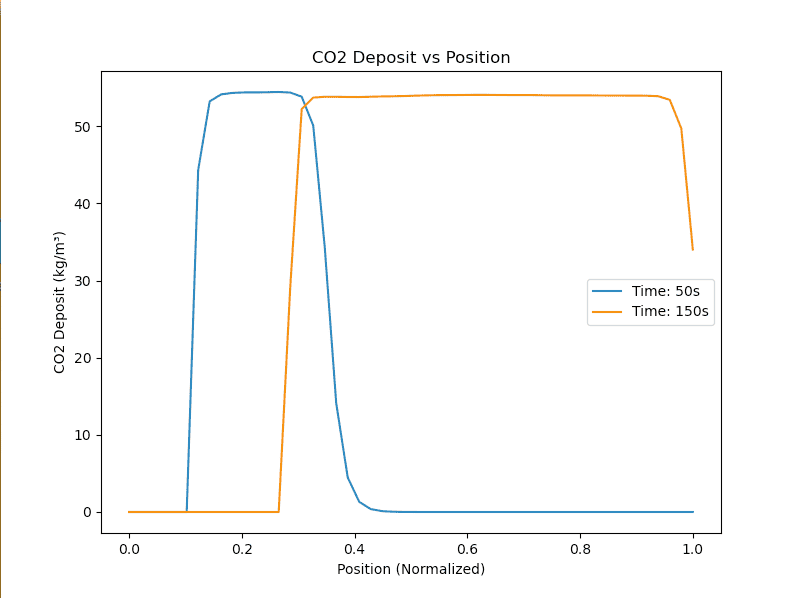H2O Deposition: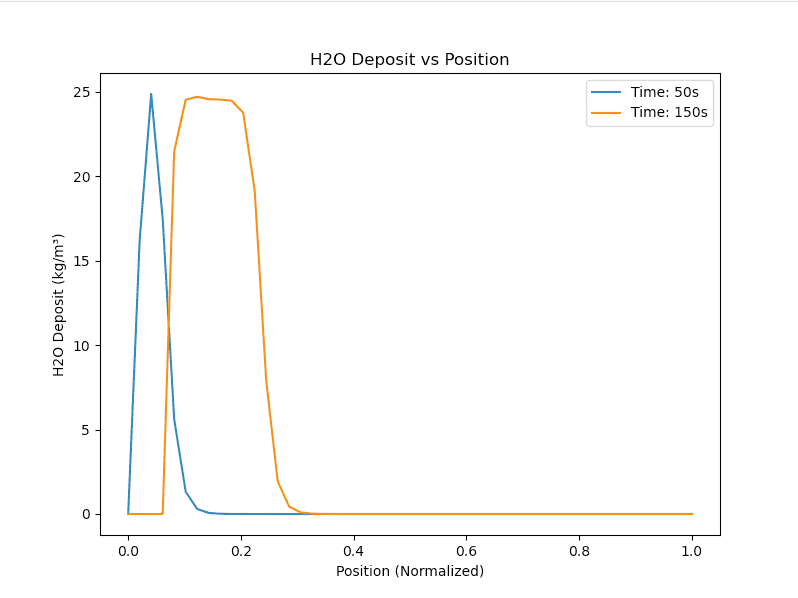Besides plotting the deposition of CO2 and H2O on the same plot, and fixing the scale units, is there anything you see here as being required in order to consider this model now "fit for use"?

I'll start defining some performance parameters for a parametric analysis if not

Here is the output after adding ##H_2O## back in. It looks good in my opinion. I ran for n=50 because n=100 was going to take too long (I'll rerun now at n=100 to get smoother plots)

Gas temperature:
View attachment 326962

CO2 deposition:
View attachment 326963

H2O Deposition:
View attachment 326965

Besides plotting the deposition of CO2 and H2O on the same plot, and fixing the scale units, is there anything you see here as being required in order to consider this model now "fit for use"?

I'll start defining some performance parameters for a parametric analysis if not
Check of overall mass balances on CO2 and H2O:: cumulative mass in = deposited mass + mass out?

Check of overall mass balances on CO2 and H2O:: cumulative mass in = deposited mass + mass out?
My apologies for the delay. It was quite difficult to extract the values of the intermediate variables (molar holdup, molar flow, molar desublimation rate, etc) from the integrator.

So we have -

Cumulative mass in:
##M_{IN,TOTAL} = \dot{m_{in}}*y_{CO_2, IN} * t\tag{1}##

The total molar amount of CO2 leaving the column up to time t is:
##\dot{m_T}## = ##\int_0^t{\dot{m}_n(t')y_{CO2}(t')dt'}##

Total molar holdup of CO2 in the gas phase:
##M_{CO2} = \sum_{j=1}^{n}(P/RT_j)y_{j,CO2}(A_cdz*\epsilon)\tag{4}##

Total molar holdup of CO2 in the solid phase:
##M_{SOLID} = \sum_{j=1}^{n}(M_j)\tag{5}##

(And the same for H2O)

Using these relationships, we get this output: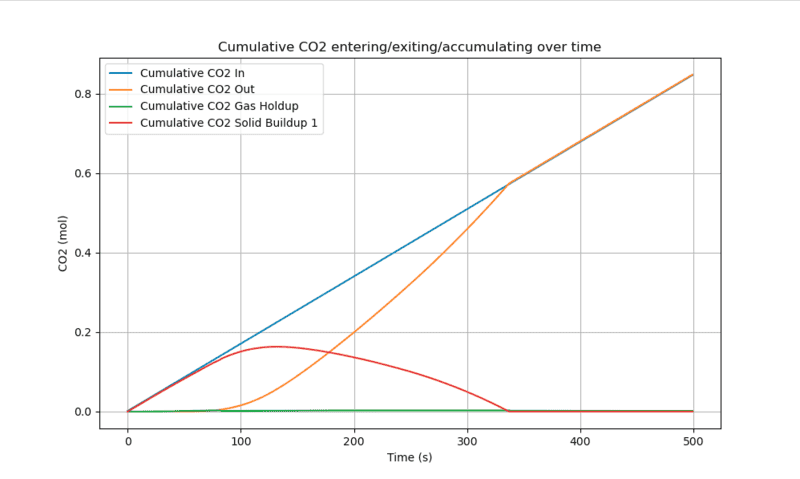At long times CO2 in = CO2 out, and at long times CO2 Gas phase molar holdup = CO2 in * number of tanks

And the same trend for H2O, except the times involved are longer as it takes H2O longer to move through the bed: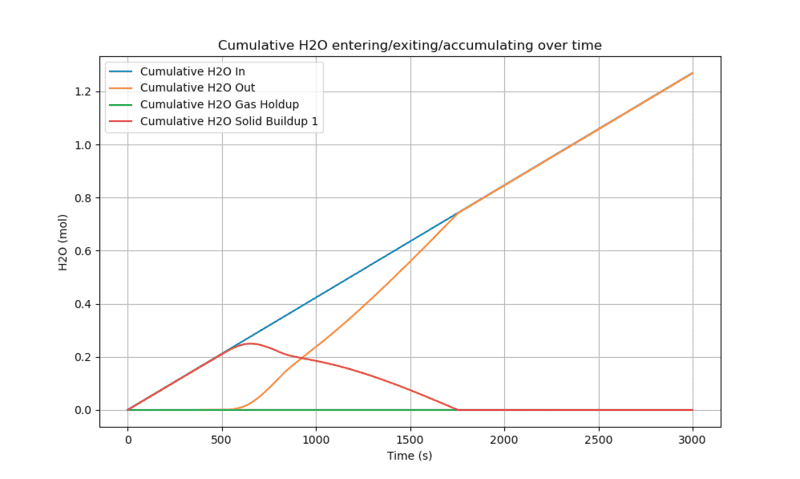If the above looks reasonable, I was hoping to define some parameters that could assess the performance of this system, before running the simulation for suitable ranges of initial/boundary conditions Possibly:

Captured Fraction:
##\eta = \frac{M_{SOLID}}{M_{IN,TOTAL}}##

In addition I was interested in defining a parameter that measures the total amount of energy required to 'capture' a given amount of CO2. This parameter would take into account the energy required to cool the bed (I should have this from the other model), and also the energy required to send combustion gas to the packed bed (via compressor). The idea being to assess different conditions versus this "energy required per unit of CO2 captured" parameter. If you think this is reasonable I'll make an initial attempt at defining that performance parameter more accurately

If you think this is reasonable I'll make an initial attempt at defining that performance parameter more accurately
Here's an inital attempt -

Assuming here that these variables are the ones we have operational control over:
1. Initial bed temperature
2. Inlet gas temperature
3. Bed pressure
4. Inlet CO2 concentration
5. Bed material

It would be useful to know which values of each of the above variables lead to the largest amount of CO2 captured. We could simply run a parametric analysis here to see that

I think it would be more useful though to know which values of each of the above variables lead to the largest amount of CO2 captured ##\textbf{per unit of energy input}## i.e. how much total energy input is required per unit mass of CO2 captured. In this packed bed system, we've got energy input in two forms:
1. Cooling of the packed bed
2. 'Pressurising' of the hot N2/CO2/H2O stream to pass it through the packed bed

Note here we have been running the model at atmospheric pressure, but we could in theory run it at any pressure up to the triple point pressure (about 5atm), which is why I included energy input #2.

So the total energy would be the sum of these (not defined properly yet).

The 'total amount of CO2 captured' could be defined as the maximum amount of CO2 captured. We have data for the amount of CO2 deposited at each point in the bed at each point in time, so we could simply take the maximum value.

Do you think the above is reasonable? If so I'll start defining the energy inputs properly. Very much open to the possibility of using other performance parameters at this stage if you think of any!